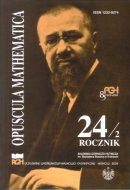Opuscula Math. 24, no. 2 (2004), 171-175

Opuscula Mathematica

# A note on a list colouring of hypergraphs

Ewa Drgas-Burchardt

Abstract. In the note we present two results. The first of them gives a sufficient condition for a colouring of a hypergraph from an assigned list. It generalises the analogous fact for graphs. The second result states that for every $$k\geq 3$$ and every $$l\geq 2$$, a distance between the list chromatic number and the chromatic number can be arbitrarily large in the class of $$k$$-uniform hypergraphs with the chromatic number bounded below by $$l$$. A similar result for $$k$$-uniform, $$2$$-colorable hypergraphs is known but the proof techniques are different.

Keywords: hypergraph, list colouring.

Mathematics Subject Classification: 05C65, 05C15.

Full text (pdf)

• Ewa Drgas-Burchardt
• University of Zielona Góra, Faculty of Mathematics Computer Science and Econometrics, ul. Podgórna 50, 65-246 Zielona Góra, Poland